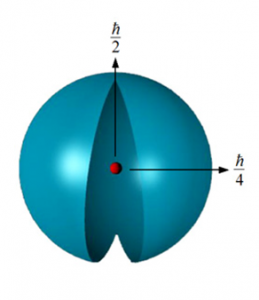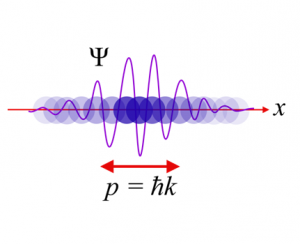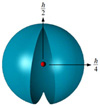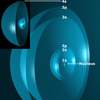# Theory Overview

Brilliant Light Power (formerly BlackLight Power) technology is built on a new classical approach to solving atoms and molecules: The Grand Unified Theory of Classical Physics (GUT-CP). This approach differs from traditional quantum mechanics, and yields many new predictions and insights.

Q: Many so-called mainstream physicists who believe in quantum theory dismiss your theory as pseudoscience. What is Mills Grand Unified Theory of Classical Physics (GUTCP) underlying the SunCell® that harnesses the new, pollution-free primary power source based on forming Hydrinos®?

The new paradigm is the realization of what was believed to be the ultimate physical reality by many of the original modern physicists such as Einstein, Schrodinger, and DeBroglie, as well as many chemists and physicists since the inception of quantum theory and the plethora of add-on theories. Mills theory is that there are NO THEORIES of physics or chemistry. There are only the simple laws of Newton’s Mechanics, Maxwell’s equations of electricity, magnetism, and electrodynamics, and the fundamental constants of nature. Laws are NOT THEORIES. They are directly confirmed, necessary and complete, and explain all physical observables on all scales. The universe is deterministic and physical wherein the underlying laws and resulting phenomena can be described by corresponding exact equations; rather than the universe being an indeterminate manifestation of probability mathematics. There is no special relativity theory, no general relativity theory, no theory of quantum mechanics, no quantum information theory, no theory of quantum electrodynamics, no extra dimensional theory, no quantum entanglement theory, no theory of Dirac mechanics, no theory of quantum chromodynamics, no Standard Model theory, no big bang theory, no inflation theory, no theory of dark energy, no string theory, no supersymmetry theory, no quantum gravity theory, no Heitler-London theory, no Hartree Fock theory, no density functional theory, no BSC theory, and so on and so on. There are no postulated principles or rules such as the Heisenberg Uncertainty Principle, Einstein Equivalence Principle of Frames of Inertia, Pauli Exclusion Principle, or Hawking Ignorance Principle or Hund’s Rule. Theorems such as the Zeno No Go Theorem are not based in reality. All observables from quarks to cosmos, spanning all 85 orders of magnitude of scale, are predicted directly by the laws of the universe in closed-form analytical expressions containing fundamental constants only.

 Hydrogen atom according to Laws of Nature is an exact physical object as shown.Hydrogen atom according to quantum theory is this mathematical expression with no physical form ψ(r,θ,ϕ) = R(r)Θ(θ)Φ(ϕ) = NRn,l(r)Plm(cos θ) eimϕQ: What is the founding principle of Mills GUT-CP?

In the atom, electrons are constantly accelerating around the proton in an atomic orbit. Yet, classical physics requires that accelerating charges radiate energy, which would cause the electron to spiral into the nucleus in a fraction of a second. This seminal problem of the stability of the atom was one of the key obstacles physicists faced early in the 20th century, and their inability to solve it led to the construction of quantum theory.

Mills solved the structure of the electron using classical physical laws, such that electron orbits were stable to radiation. This allowed Mills to construct a new theory of atoms and molecules that was based entirely on classical physics. And unlike other attempts in the 20th century, the result was not merely a “hidden-variables” reinterpretation of the quantum formalism, but a ground-up reconstruction of atomic theory.

This new model of the atom predicted the theoretical existence of the hydrino, or energy states of the hydrogen atom that exist below the -13.6 eV energy state of atomic hydrogen that is the basis of Brilliant Light Power’s power source.Q: How does the GUT-CP describe nature? According to Mills GUT-CP, nature is classical. Electrons, when bound in an atom, are considered to be discrete two-dimensional spherical membranes of charge and current that completely surround the nucleus as a bubble. These shells, called electron atomic orbitals, each have an organized pattern of super-current filaments on the surface that gives rise to electron spin. The current may be modulated with a time and spherical harmonic pattern that gives rise to orbital angular momentum. Electrons obey classical physics such that the intrinsic electron angular momentum that arises from the pattern of motion is quantized, and invoking this property predicts that bound electrons are stable to emitting radiation according to Maxwellian electrodynamics. This solves the problem that has plagued atomic physics since the Bohr model of the atom: how an electron, continuously accelerating in the Coulombic field of the proton, is able to remain in a stable orbit. This approach is extended to solve multi-electron atoms and molecules. In multi-electron atoms, bound electrons group into a series of concentric shells, each of which is an atomic orbital and may contain several electrons. In molecules, the electrons stretch over two nuclei to form a prolate spheroidal shell with the nuclei at the foci. Each reaches an equipotential, minimum energy configuration for the system, governed by Maxwellian and Newtonian laws. From within this frame of reference, GUT-CP unwinds the mysteries of quantum theory. GUT-CP is not a “hidden variables” interpretation of the formalism of quantum theory, rather, it is a new classical theory based on Maxwell’s Equations and Newton’s Laws. It explains canonical experiments of quantum mechanics such as the double-slit experiments and the Aspect experiments classically.

Q: What does the GUT-CP predict? How has it been validated?

Mills GUT-CP is a confirmable theory, meaning it makes many claims about nature that can be confirmed or denied through experiment. The GUT-CP predicts thousands of atomic and molecular parameters that match known data to high accuracy, often to much higher accuracy than predictions by quantum mechanics. Further, several data sets produced by the GUT-CP have never been calculated before.

Data sets include the electron spin, g-factor, ionization energies of 1-20 electron atoms and ions (400 states); the state lifetimes and line intensities of hydrogen; the excited states of helium; the excited states of H2, the relationships between the masses of fundamental particles; the bond distances, energies, angles, and dipole moments of over 800 molecules; and the parameters of a variety of extended solids. From the molecular theory, Brilliant Light Power has launched a molecular modeling subsidiary, Millsian Inc.

The GUT-CP also predicted some phenomena new to science. Before 1995, it predicted that the universe was accelerating in its expansion, and predicted the rate of acceleration, which was confirmed experimentally later that decade, much to the surprise of the scientific community. The GUT-CP also correctly predicted the mass of the top quark and the lack of time dilation in highly redshifted quasars.

The GUT-CP also predicts the existence of hydrinos: each hydrino atom being a stable energy state of the hydrogen atom wherein the principle quantum number of the Rydberg formula of atomic hydrogen excited states is replaced by a reciprocal integer. Thus, these exist below the first nonradiative n = 1 state, erroneously called the “ground state” by quantum theory. Hydrinos further react to form the corresponding very stable molecules, and neither hydrinos nor molecular hydrinos emit or absorb electromagnetic radiation as is typical of electronic transitions of the ordinary species. Observations support that hydrinos is the identity of the dark matter of the universe. Brilliant Light Power is engaged in experimentally characterizing hydrinos and developing the technology for commercial use.

See the Molecular and Atomic Physics Summary Tables for predicted vs. observed values. See a list of theoretical papers published in journals, or visit our technical papers page.

Q: What is the essence of GUTCP?

The essence of GUTCP is that every solution is for the true or real source of the observable wherein any solution of an observable as the inherent information for all observables that can be related to that observable. GUTCP is not physically baseless mathematics that outputs an isolated meaningless number. Rather is provides the exact solution of physical reality: what an electron, atom, molecule, or other aspect of the universe including the total of the universe is in essence.

As a specific comparison, quantum mechanics can and GUTCP both provide results for atomic ionization energy, but the quantum mechanical result is just a number that is not property of the actual solution for each atom. The GUTCP actually solves the atom. More importantly, every electron of an atom or every atomic and molecular-bond electron of a molecule of boundless extent and complexity are solved exactly by GUTCP. For example, consider Figure 5.3 of the GUTCP showing the solution of ALL 19 electrons of K. That solution gives the successive ionization of K, K+, K2+, K3+, K4+, K5+, K6+, K7+, K8+, K9+, K10+, K11+, K12+, K13+, K14+, K15+, K16+, K17+, and K18+. GUTCP exactly solves atoms, molecules, particles, …the universe. It solves all aspects of an entity of nature. QM is just kludged mathematics adjusted to give a single meaningless number.

Moreover, the ionization energies of ALL atoms and ions of a given number of electrons is a closed-form analytical equation containing the nuclear charge and fundamental constants only: no adjustable parameters, all based on physical laws. For example, consider each successive electron of K: K, K+, K2+, K3+, K4+, K5+, K6+, K7+, K8+, K9+, K10+, K11+, K12+, K13+, K14+, K15+, K16+, K17+, and K18+. Each solution is a specific solution of the general solution of ALL atoms and ions having the same number of electrons.

Since the exact solutions for ionization energies are the actual solution of the atoms and ions, GUTCP uses them in myriad other closed-form solutions having fundamental constants only such as electron scattering from the helium atom, excited states of atomic helium and helium ion as well as superfluid helium and helium condensate. As further examples, the atomic solutions are used for the basis of the solutions of thousands of molecules that can be extended to an infinite number of boundless complexity including bulk compositions of matter such as graphene, graphite, diamond, and fullerene, all exact solutions, based on physical laws only. Quantum mechanics has never solved a single physical entity, gives only a meaningless number.

Moreover, the solution of potassium atom for example further solves ionic potassium compounds and gives the exact solution of potassium metal. Quantum mechanics is worthless in this regard even at generating a meaningless number.

A fundamental, most encompassing aspect is that GUTCP uses the light-speed case of the angular-frequency energy, the electric energy, and the magnetic energy solutions of atomic electrons to solve the masses of all fundamental particles, the origin of gravity, and the cosmological parameters of the universe including predicting the acceleration of the expansion of the cosmos before it was observed. Using proven classical physical laws, GUTCP solves quarks to cosmos uniquely, 85 orders of magnitude of scale, using the same analytical equations containing fundamental constants only. In contrast, quantum mechanics gives infinity of possible kludged purely mathematical algorithms to force a single meaningless number in each application having no connection to any other meaningless number in another.

Q: What is the response to criticism that the GUT-CP function that describes the radial aspect of the electron is a Dirac delta function that is not a solution of the Schrodinger equation?

Some theoreticians have objected to the treatment of both the Green’s function and a Dirac delta function regarding the GUT-CP solution of electron structure. It is well known that the Green’s function and a Dirac delta function regarding electromagnetic waves are pillars of classical electrodynamics. Both of these functions are commonly used in classical physics to solve general inhomogeneous equations, and describe radiation from source currents that behave as point sources, but are not necessarily a point source due to the source current distribution in space and time. The GUT-CP applies the Green’s function as a generally accepted method to solve a partial differential equation, e.g. the Helmholtz equation for the nature of electromagnetic radiation. Moreover, it is a presumptive error that the GUT-CP’s classical solution of the bound electron requires that a Dirac delta function be a solution of the three dimensional wave equation or the Schrodinger equation. The GUT-CP does not solve the physical nature of the electron by postulating a wave operator for which the electron probability density must be a solution. Rather, it is held that the energy levels of the bound electron change by absorption and emission of photons that comprise quanta of electromagnetic waves that obey the classical wave equation. These waves are matched to the electron as the source current, and the electron current density is solved correctly as comprising a radial Dirac delta function and spherical harmonic angular and harmonic temporal functions wherein the non-excited state is non-radiative. The results based on the solution of the electron structure from proven classical physical laws match observations for all observables such as the principal energy levels, spectral splitting in a magnetic field, orbital and spin angular momentum and spin-orbit coupling, Lamb shift, fine structure, hyperfine structure, Stern-Gerlach experiment, g factor, state lifetimes, and Einstein A coefficients. This is not the case for the untenable results of quantum mechanics regarding a singularity with all possible energies and positions simultaneously.

Q: Do hydrinos violate conservation of energy?

No. The hydrogen atom is stable to emitting a photon directly. But, an unstable hydrogen state forms when a hydrogen atom resonantly and nonradiative exchanges energy with another atom (a catalyst) capable of accepting energy in multiples of 27.2 eV. This process relies on multipole coupling between the hydrogen atom and the catalyst. The unstable state then emits further energy as radiation of a characteristic continuum profile as the electron drops into a lower energy state, in which the electron is closer to the proton, forming a higher binding energy hydrogen atom. Energy is conserved in this process. The resulting hydrino atom cannot be converted into a normal hydrogen atom without the addition of collisional energy to elevate the electron’s energy by at least that released in the formation of the hydrino.